SSC (English Medium) Class 10th Board ExamMaharashtra State Board
Share

Balbharati solutions for Class 10th Board Exam Geometry chapter 2 - Pythagoras Theorem

Textbook for SSC Class 10 Mathematics 2

Balbharati SSC Class 10 Mathematics 2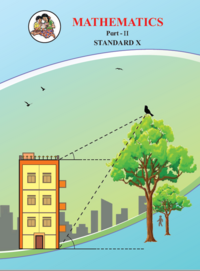Chapter 2: Pythagoras Theorem

Chapter 2: Pythagoras Theorem solutions [Pages 38 - 39]

Q 1.1 | Page 38

Identify, with reason, which of the following are Pythagorean triplets.
(3, 5, 4)

Q 1.2 | Page 38

Identify, with reason, which of the following are Pythagorean triplets.(4, 9, 12)

Q 1.3 | Page 38

Identify, with reason, which of the following are Pythagorean triplets.

(5, 12, 13)

Q 1.4 | Page 38

Identify, with reason, which of the following are Pythagorean triplets.

(24, 70, 74)

Q 1.5 | Page 38

Identify, with reason, which of the following are Pythagorean triplets.

(10, 24, 27)

Q 1.6 | Page 38

Identify, with reason, which of the following are Pythagorean triplets.(11, 60, 61)

Q 4 | Page 39

In the given figure. Find RP and PS using the information given in ∆PSR.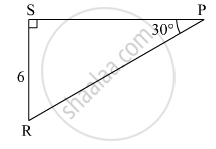Q 5 | Page 39

For finding AB and BC with the help of information given in the figure, complete following activity.

AB = BC ..........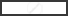$\therefore \angle BAC =$$\therefore AB = BC =$$\times AC$

$=$$\times \sqrt{8}$

$=$$\times 2\sqrt{2}$

=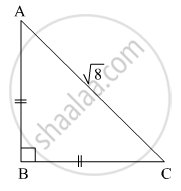Q 6 | Page 39

Find the side and perimeter of a square whose diagonal is 10 cm ?

Q 7 | Page 39

In the given figure, ∠DFE = 90°, FG ⊥ ED, If GD = 8, FG = 12, find (1) EG (2) FD and (3) EF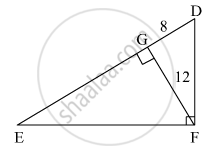Q 8 | Page 39

Find the diagonal of a rectangle whose length is 35 cm and breadth is 12 cm.

Q 9 | Page 39

In the given figure, M is the midpoint of QR. ∠PRQ = 90°. Prove that, PQ= 4PM– 3PR2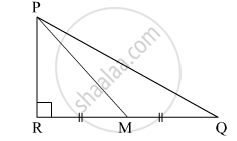Q 10 | Page 39

Walls of two buildings on either side of a street are parellel to each other. A ladder 5.8 m long is placed on the street such that its top just reaches the window of a building at the height of 4 m. On turning the ladder over to the other side of the street , its top touches the window of the other building at a height 4.2 m. Find the width of the street.

Chapter 2: Pythagoras Theorem solutions [Page 43]

Q 1 | Page 43

In ∆PQR, point S is the midpoint of side QR. If PQ = 11, PR = 17, PS =13, find QR.

Q 2 | Page 43

In ∆ABC, AB = 10, AC = 7, BC = 9 then find the length of the median drawn from point C to side AB

Q 3 | Page 43

In the given figure seg PS is the median of ∆PQR and PT ⊥ QR. Prove that,

${PR}^2 = {PS}^2 + QR \times ST + \left( \frac{QR}{2} \right)^2$

${PQ}^2 = {PS}^2 - QR \times ST + \left( \frac{QR}{2} \right)^2$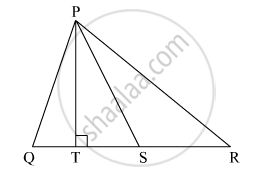Q 4 | Page 43

In ∆ABC, point M is the midpoint of side BC.
If, AB+ AC= 290 cm2, AM = 8 cm, find BC.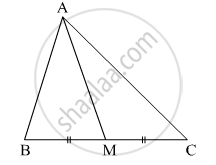Q 5 | Page 43

In the given figure, point T is in the interior of rectangle PQRS, Prove that, TS+ TQ= TP+ TR(As shown in the figure, draw seg AB || side SR and A-T-B)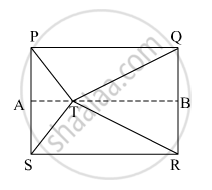Chapter 2: Pythagoras Theorem solutions [Pages 43 - 46]

Q 1.1 | Page 43

Some questions and their alternative answers are given. Select the correct alternative.
(1) Out of the following which is the Pythagorean triplet?

(A) (1, 5, 10) (B) (3, 4, 5) (C) (2, 2, 2) (D) (5, 5, 2)
Q 1.2 | Page 43

In a right angled triangle, if sum of the squares of the sides making right angle is 169 then what is the length of the hypotenuse?

(A) 15 (B) 13 (C) 5 (D) 12
• (A) 15

• (B) 13

• (C) 5

• (D) 12

Q 1.3 | Page 43

Out of the dates given below which date constitutes a Pythagorean triplet ?

• (A) 15/08/17

• (B) 16/08/16

• (C) 3/5/17

• (D) 4/9/15

Q 1.4 | Page 43

If a, b, c are sides of a triangle and a+ b= c2, name the type of triangle.

• (A) Obtuse angled triangle

• (B) Acute angled triangle

• (C) Right angled triangle

• (D) Equilateral triangle

Q 1.5 | Page 43

Find perimeter of a square if its diagonal is $10\sqrt{2}$

(A)10 cm (B) $40\sqrt{2}$cm (C) 20 cm (D) 40 cm

• (A)10 cm

• (B) $40\sqrt{2}$cm

• (C) 20 cm

• (D) 40 cm

Q 1.6 | Page 43

Altitude on the hypotenuse of a right angled triangle divides it in two parts of lengths 4 cm and 9 cm. Find the length of the altitude.

• (A) 9 cm

• (B) 4 cm

• (C) 6 cm $2\sqrt{6}$

• (D) cm

Q 1.7 | Page 43

Height and base of a right angled triangle are 24 cm and 18 cm find the length of its hypotenuse

• (A) 24 cm

• (B) 30 cm

• (C) 15 cm

• (D) 18 cm

Q 1.8 | Page 43

In ∆ABC, AB = $6\sqrt{3}$ cm, AC = 12 cm, BC = 6 cm. Find measure of ∠A.

• (A) 30°

• (B) 60°

• (C) 90°

• (D) 45°

Q 2.1 | Page 44

Solve the following example.

Find the height of an equilateral triangle having side 2a.

Q 2.2 | Page 44

Do sides 7 cm, 24 cm, 25 cm form a right angled triangle ? Give reason

Q 2.3 | Page 44

Find the length a diagonal of a rectangle having sides 11 cm and 60 cm.

Q 2.4 | Page 44

Find the length of the hypotenuse of a right angled triangle if remaining sides are 9 cm and 12 cm.

Q 2.5 | Page 44

In ∆PQR; PQ =$\sqrt{8}$, QR =$\sqrt{5}$, PR = $\sqrt{3}$. Is ∆PQR a right angled triangle ? If yes, which angle is of 90°?

Q 2.5 | Page 44

A side of an isosceles right angled triangle is x. Find its hypotenuse.

Q 3 | Page 44

In ∆RST, ∠S = 90°, ∠T = 30°, RT = 12 cm then find RS and ST.

Q 4 | Page 44

Find the diagonal of a rectangle whose length is 16 cm and area is 192 sq.cm ?

Q 5 | Page 44

Find the length of the side and perimeter of an equilateral triangle whose height is $\sqrt{3}$ cm.

Q 6 | Page 44

In ∆ABC seg AP is a median. If BC = 18, AB2 + AC2 = 260 Find AP.

Q 7 | Page 45

∆ABC is an equilateral triangle. Point P is on base BC such that PC = $\frac{1}{3}$ BC, if AB = 6 cm find AP.

Q 8 | Page 45

From the information given in the figure, prove that PM = PN =  $\sqrt{3}$  × a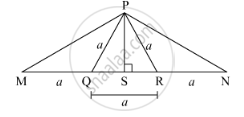Q 9 | Page 45

Prove that the sum of the squares of the diagonals of a parallelogram is equal to the sum of the squares of its sides.

Q 10 | Page 45

Pranali and Prasad started walking to the East and to the North respectively, from the same point and at the same speed. After 2 hours distance between them was $15\sqrt{2}$

km. Find their speed per hour.

Q 11 | Page 45

In ∆ABC, ∠BAC = 90°, seg BL and seg CM are medians of ∆ABC. Then prove that:
4(BL+ CM2) = 5 BC2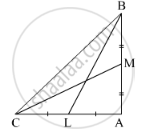Q 12 | Page 45

Sum of the squares of adjacent sides of a parallelogram is 130 sq.cm and length of one of its diagonals is 14 cm. Find the length of the other diagonal.

Q 13 | Page 45

In ∆ABC, seg AD ⊥ seg BC DB = 3CD. Prove that :
2AB= 2AC+ BC2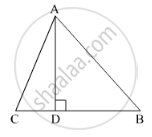Q 14 | Page 44

In an isosceles triangle, length of the congruent sides is 13 cm and its base is 10 cm. Find the distance between the vertex opposite the base and the centroid.

Q 15 | Page 46

In a trapezium ABCD, seg AB || seg DC seg BD ⊥ seg AD, seg AC ⊥ seg BC, If AD = 15, BC = 15 and AB = 25. Find A(▢ABCD)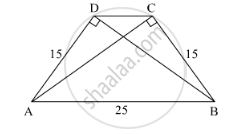Q 16 | Page 44

In the given figure, ∆PQR is an equilateral triangle. Point S is on seg QR such thatn QS =n$\frac{1}{3}$ QR.

Prove that : 9 PS= 7 PQ2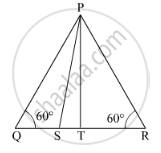Q 17 | Page 44

Seg PM is a median of ∆PQR. If PQ = 40, PR = 42 and PM = 29, find QR.

Q 18 | Page 46

Seg AM is a median of ∆ABC. If AB = 22, AC = 34, BC = 24, find AM

Chapter 2: Pythagoras Theorem

Balbharati SSC Class 10 Mathematics 2Balbharati solutions for Class 10th Board Exam Geometry chapter 2 - Pythagoras Theorem

Balbharati solutions for Class 10th Board Exam Geometry chapter 2 (Pythagoras Theorem) include all questions with solution and detail explanation. This will clear students doubts about any question and improve application skills while preparing for board exams. The detailed, step-by-step solutions will help you understand the concepts better and clear your confusions, if any. Shaalaa.com has the Maharashtra State Board Textbook for SSC Class 10 Mathematics 2 solutions in a manner that help students grasp basic concepts better and faster.

Further, we at Shaalaa.com are providing such solutions so that students can prepare for written exams. Balbharati textbook solutions can be a core help for self-study and acts as a perfect self-help guidance for students.

Concepts covered in Class 10th Board Exam Geometry chapter 2 Pythagoras Theorem are Apollonius Theorem, Application of Pythagoras Theorem in Acute Angle and Obtuse Angle, Converse of Pythagoras Theorem, Pythagoras Theorem, Theorem of geometric mean, Similarity in Right Angled Triangles, 30 - 60 - 90 and 45 - 45 - 90 Theorem.

Using Balbharati Class 10th Board Exam solutions Pythagoras Theorem exercise by students are an easy way to prepare for the exams, as they involve solutions arranged chapter-wise also page wise. The questions involved in Balbharati Solutions are important questions that can be asked in the final exam. Maximum students of Maharashtra State Board Class 10th Board Exam prefer Balbharati Textbook Solutions to score more in exam.

Get the free view of chapter 2 Pythagoras Theorem Class 10th Board Exam extra questions for Geometry and can use Shaalaa.com to keep it handy for your exam preparation

S